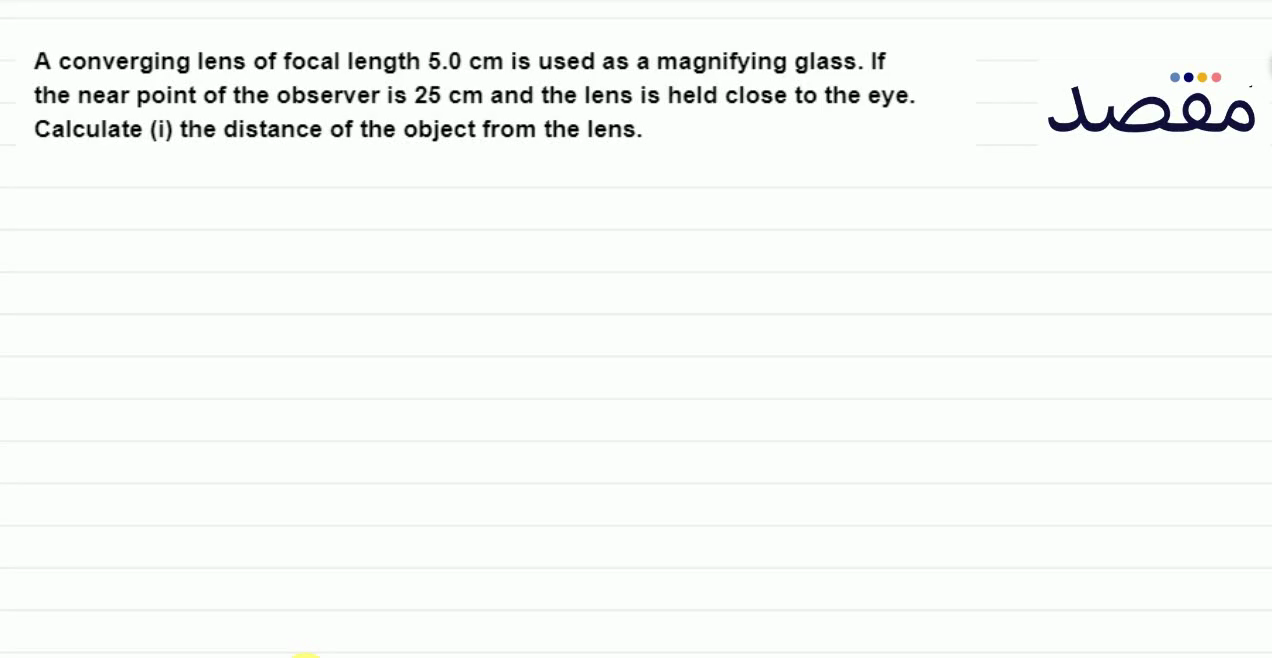# Classes

Change the way you learn with Maqsad's classes. Local examples, engaging animations, and instant video solutions keep you on your toes and make learning fun like never before!

Class 9Class 10First YearSecond Year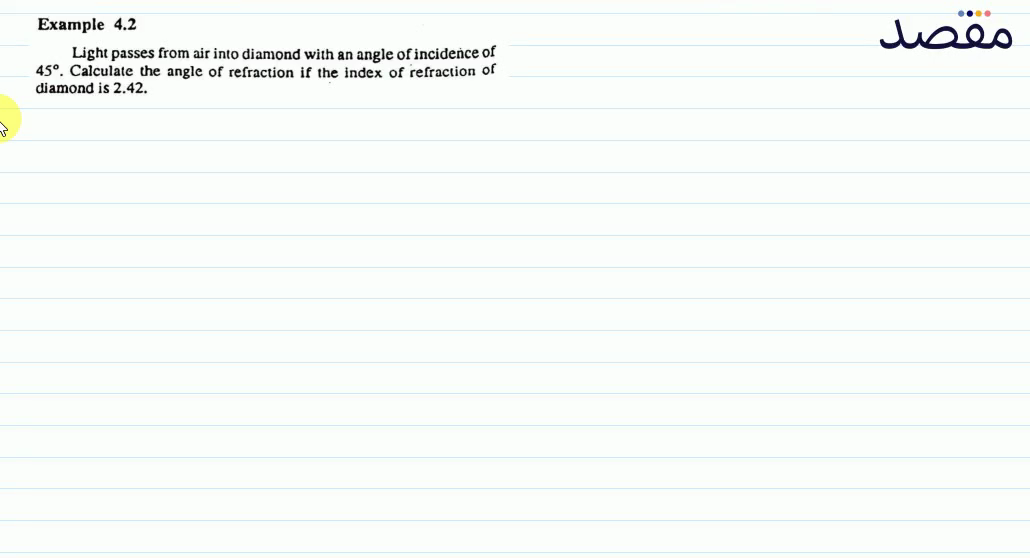### Example 4.2 Light passes from air into diamond with an angle of incidence of 45^{\circ} . Calculate the angle of refraction if the index of refraction of diamond is 2.42 .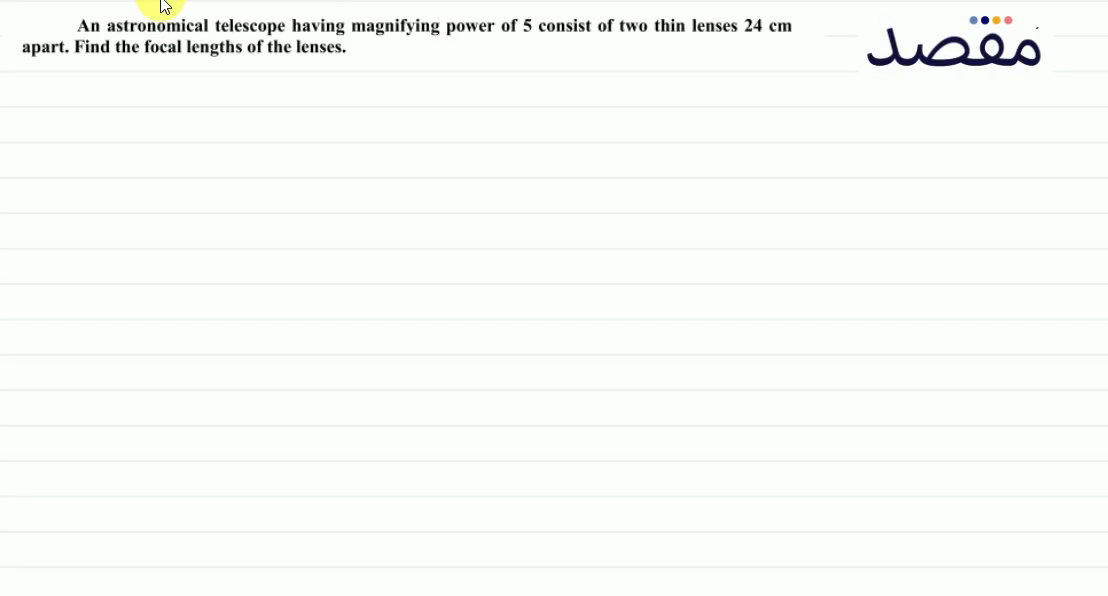### An astronomical telescope having magnifying power of 5 consist of two thin lenses 24 \mathrm{~cm} apart. Find the focal lengths of the lenses.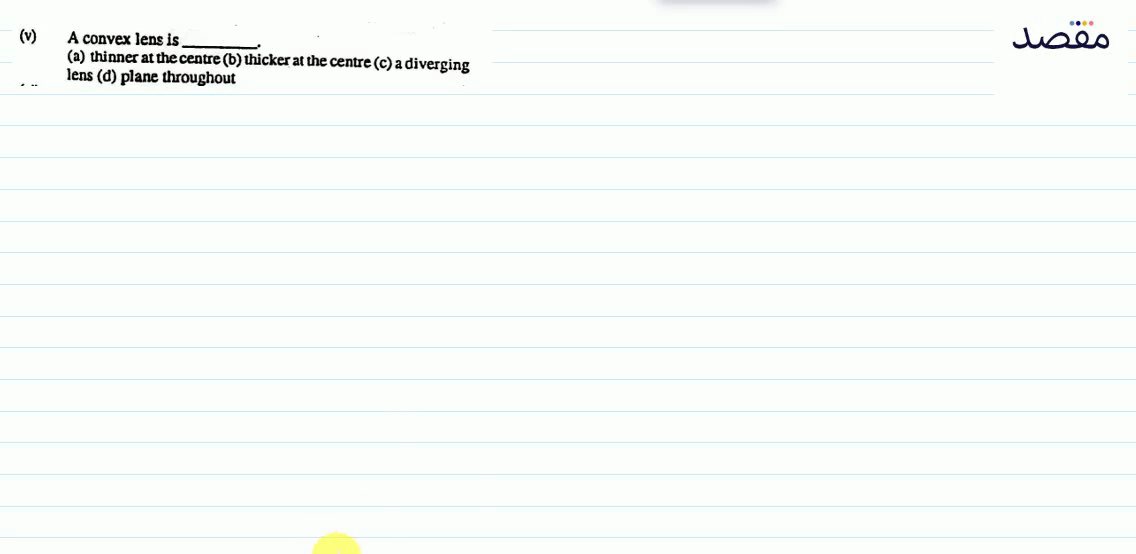### (v) A convex lens is(a) thinner at the centre (b) thicker at the centre (c) a diverging lens (d) plane throughout### 4.2 The speed of light in water is 2.25 \times 10^{5} \mathrm{~km} / \mathrm{s} . What is the index of refraction of water?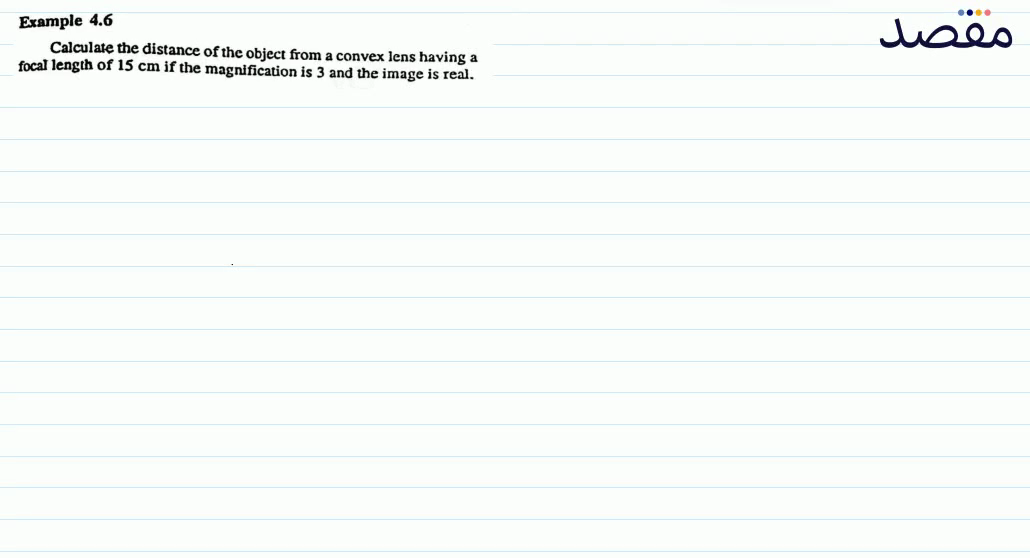### Example 4.6 Calculate the distance of the object from a convex lens having a focal length of 15 \mathrm{~cm} if the magnification is 3 and the image is real.